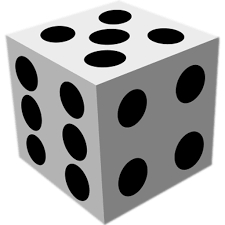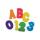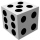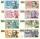# The dice

Find the probability that a number divisible by two or three will fall when the dice are rolled.

p =  0.6667

### Step-by-step explanation:Did you find an error or inaccuracy? Feel free to write us. Thank you!Tips to related online calculators
Would you like to compute count of combinations?

## Related math problems and questions:

• Divisible by nineHow many three-digit natural numbers in total are divisible without a remainder by the number 9?
• Fall sum or sameFind the probability that if you roll two dice, it will fall the sum of 10, or the same number will fall on both dice.
• Divisibility by 12Replace the letters A and B by digits so that the resulting number x is divisible by twelve /find all options/. x = 2A3B How many are the overall solutions?
• Find unknown numberWhat is the number between 50 and 55 that is divisible by 2,3,6,9?
• By sixFrom the digits 1,2,3,4 we create the long integer number 123412341234. .. .. , which will have 962 digits. Is this number divisible by 6?
• NumberWhat number should be placed instead of the asterisk in number 702*8 to get a number divisible by 6?
• Sum or productWhat is the probability that two dice fall will have the sum 7 or product 12?
• Roll the diceWhat is the probability that if we roll the dice, a number less than 5 falls?
• Divisible by fourAre 240 divisible by 4?
• Double six-sixWhat is the probability, that a player in the games of ludo will throw a double 6 twice .
• Six on diceWhat is the probability that when throwing two dice will fall at least one six?
• Seven numbersWrite seven 4-digit numbers that are divisible by 3 and at the same time by 4.
• What are 3What are the two digits which when inserted in the blank spaces will make 234 _ _ divisible by 8?
• Two-digit numberI am a two-digit number less than 20. When I divide by three, then you get the rest one, and when you divide me by four, you get also rest 1. What number am I?
• Air draftThe numbers 1,2,3,4,5 are written on five tickets on the table. Air draft randomly shuffled the tickets and composed a 5-digit number from them. What is the probability that he passed: and, the largest possible number b, the smallest possible number c, a
• One diceCalculate the probability of one dice roll with the numbers 1, 2, 3, 4, 5, 6 on the walls. Write the results in a notebook in the shape of a fraction in the basic form: 2/3. a, The number 1 falls on the cube. b, The number 5 falls on the cube. c, An even
• Cash backAfter a trip 24 students have left more than 650 but less than 690 CZK (Kc). How much each student receives return when the amount is divisible by the number of pupils?